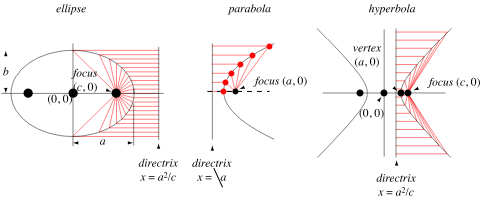# GET TUTORING NEAR ME!(800) 434-2582

/ Get Math Help

## Directrix

IllustrationAlternate name
Definition

The directrix of a conic section is the line which, together with the point known as the focus, serves to define a conic section as the locus of points whose distance from the focus is proportional to the horizontal distance from the directrix, with r being the constant of proportionality. If the ratio r = 1, the conic is a parabola, if r<1, it is an ellipse, and if r>1, it is a hyperbola. Hyperbolas and noncircular ellipses have two distinct foci and two associated directrices, each directrix being perpendicular to the line joining the two foci.

Related terms